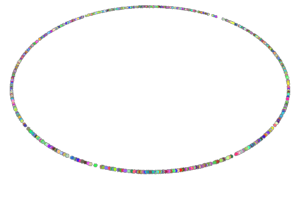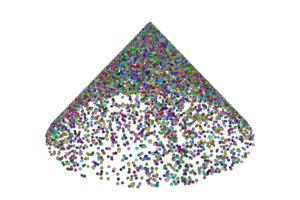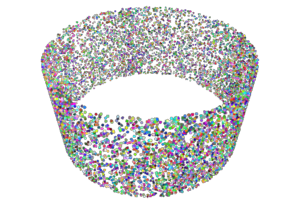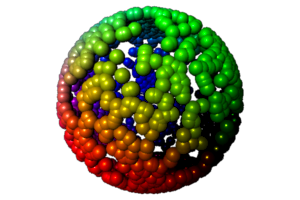### RenderMan Procedural PrimitivesRandomness

#### Introduction

Being able to generate points distributed randomly within or over a surface can be useful when modeling phenomena such as fireworks. This tutorial presents in listings 1 to 9 some useful utility procs implemented in Python, Tcl and the 'C' programming language. The implementations of the utility procs will be given followed by examples of their use.

#### Proc randBetween

This proc returns a random value between two input values. Although it is a very simple it is surprisingly useful for positioning objects, such as RenderMan points and curves, as well as setting randomized rgb components of colors.

Listing 1 - Python Implementation

 ```import random def randBetween(min, max): return random.random() * (max - min) + min```

Listing 2 - Tcl Implementation

 ```proc randBetween { min max } { return [expr rand() * (\$max - \$min) + \$min] }```

Listing 3 - 'C' Implementation

 ```#include double randBetween(double min, double max) { return ((double)rand()/RAND_MAX) * (max - min) + min; }```

#### Procs length & normalize

The proc `length` returns the length of a vector. The proc `normalize` normalizes a vector. Typically, the input values to this proc are the xyz position of a geometric point. However, the location of the geometric point can be considered to represent the "head" of a vector and as such it can be converted to a unit vector.

Listing 4 - Python Implementation

 ```import math def length(x, y, z): return math.sqrt(x*x + y*y + z*z) def normalize(x, y, z): len = length(x, y, z) return x/len, y/len, z/len```

Listing 5 - Tcl Implementation

 ```proc length { x y z } { return [expr sqrt(\$x*\$x + \$y*\$y + \$z*\$z)] } proc normalize { x y z } { set len [length \$x \$y \$z] return [list [expr \$x/\$len] [expr \$y/\$len] [expr \$z/\$len]] }```

Listing 6 - 'C' Implementation

 ```#include #include double length(double pnt) { return sqrt((pnt * pnt) + (pnt * pnt) + (pnt * pnt)); } void normalize(double pnt) { double len = length(pnt); pnt /= len; pnt /= len; pnt /= len; }```

#### Proc scaleVector

This proc returns the xyz values of a vector re-sized to a specified length.

Listing 7 - Python Implementation

 ```def scaleVector(x, y, z, sc): return x*sc, y*sc, z*sc ```

Listing 8 - Tcl Implementation

 ```proc scaleVector { vect sc } { set X [expr [lindex \$vect 0] * \$sc] set Y [expr [lindex \$vect 1] * \$sc] set Z [expr [lindex \$vect 2] * \$sc] return [list \$X \$Y \$Z] }```

Listing 9 - 'C' Implementation

 ```void scaleVector(double pnt, double sc) { pnt *= sc; pnt *= sc; pnt *= sc; }```

#### Examples of Use

This section provides some simple examples of how the procs given in listing 1 to 9 can be used with RenderMan's point primitive. For brevity, the examples are only given in Python.

##### RiPoints in a Rectangular VolumeFigure 1

Listing 10 - rectangular box

 ```import sys, math, random def box(width, height, depth, num, size): print 'Points \"P\" [' for n in range(num): x = randBetween(-width/2, width/2) y = randBetween(-height/2, height/2) z = randBetween(-depth/2, depth/2) print '%s %s %s' % (x, y, z) print '] \"constantwidth\" [%s]' % size print '\"Cs\" [' for n in range(num): r = randBetween(0, 1) g = randBetween(0, 1) b = randBetween(0, 1) print '%s %s %s' % (r, g, b) print ']' def main(): args = sys.stdin.readline() while args: arg = args.split() pixels = float(arg) width = float(arg) height = float(arg) depth = float(arg) num = int(arg) size = float(arg) print 'TransformBegin' box(width, height, depth, num, size) print 'TransformEnd' sys.stdout.write('\377') sys.stdout.flush() # read the next set of inputs args = sys.stdin.readline() if __name__ == "__main__": main() ```

##### RiPoints in a RingFigure 2

Listing 11 - Ring

 ```import sys, math, random def ring(rad, num, size): print 'Points \"P\" [' for n in range(num): x = randBetween(-1, 1) y = 0 z = randBetween(-1, 1) x,y,z = normalize(x,y,z) x,y,z = scaleVector(x,y,z,rad) print '%s %s %s' % (x, y, z) print '] \"constantwidth\" [%s]' % size print '\"Cs\" [' for n in range(num): r = randBetween(0, 1) g = randBetween(0, 1) b = randBetween(0, 1) print '%s %s %s' % (r, g, b) print ']' def main(): args = sys.stdin.readline() while args: arg = args.split() pixels = float(arg) rad = float(arg) num = int(arg) size = float(arg) print 'TransformBegin' ring(rad, num, size) print 'TransformEnd' sys.stdout.write('\377') sys.stdout.flush() # read the next set of inputs args = sys.stdin.readline() if __name__ == "__main__": main()```

##### RiPoints on a DiskFigure 3

Listing 12 - Disk

 ```import sys, math, random def disk(rad, num, size): print 'Points \"P\" [' for n in range(num): x = randBetween(-1, 1) y = 0 z = randBetween(-1, 1) x,y,z = normalize(x,y,z) randRadius = randBetween(0, rad) x,y,z = scaleVector(x,y,z,randRadius) print '%s %s %s' % (x, y, z) print '] \"constantwidth\" [%s]' % size print '\"Cs\" [' for n in range(num): r = randBetween(0, 1) g = randBetween(0, 1) b = randBetween(0, 1) print '%s %s %s' % (r, g, b) print ']' def main(): args = sys.stdin.readline() while args: arg = args.split() pixels = float(arg) rad = float(arg) num = int(arg) size = float(arg) print 'TransformBegin' disk(rad, num, size) print 'TransformEnd' sys.stdout.write('\377') sys.stdout.flush() # read the next set of inputs args = sys.stdin.readline() if __name__ == "__main__": main() ```

##### RiPoints on a ConeFigure 4

Listing 13 - Cone

 ```import sys, math, random def cone(rad, num, size): print 'Points \"P\" [' for n in range(num): x = randBetween(-1, 1) y = 0 z = randBetween(-1, 1) x,y,z = normalize(x,y,z) randRadius = randBetween(0, rad) x,y,z = scaleVector(x,y,z,randRadius) y += 1 - randRadius # <<--- print '%s %s %s' % (x, y, z) print '] \"constantwidth\" [%s]' % size print '\"Cs\" [' for n in range(num): r = randBetween(0, 1) g = randBetween(0, 1) b = randBetween(0, 1) print '%s %s %s' % (r, g, b) print ']' def main(): args = sys.stdin.readline() while args: arg = args.split() pixels = float(arg) rad = float(arg) num = int(arg) size = float(arg) print 'TransformBegin' cone(rad, num, size) print 'TransformEnd' sys.stdout.write('\377') sys.stdout.flush() # read the next set of inputs args = sys.stdin.readline() if __name__ == "__main__": main() ```

##### RiPoints on a CylinderFigure 4

Listing 14 - Cylinder

 ```import sys, math, random def cylinder(rad, depth, height, num, size): print 'Points \"P\" [' for n in range(num): x = randBetween(-1, 1) y = 0 z = randBetween(-1, 1) x,y,z = normalize(x,y,z) y = randBetween(depth, height) # <<--- print '%s %s %s' % (x, y, z) print '] \"constantwidth\" [%s]' % size print '\"Cs\" [' for n in range(num): r = randBetween(0, 1) g = randBetween(0, 1) b = randBetween(0, 1) print '%s %s %s' % (r, g, b) print ']' def main(): args = sys.stdin.readline() while args: arg = args.split() pixels = float(arg) rad = float(arg) depth = float(arg) height = float(arg) num = int(arg) size = float(arg) print 'TransformBegin' cylinder(rad, depth, height, num, size) print 'TransformEnd' sys.stdout.write('\377') sys.stdout.flush() # read the next set of inputs args = sys.stdin.readline() if __name__ == "__main__": main() ```

##### Spheres on a SphereFigure 5

Listing 15 - Sphere

 ```import sys, math, random def spheres(rad, num, size): for n in range(num): x = randBetween(-1.0, 1.0) y = randBetween(-1.0, 1.0) z = randBetween(-1.0, 1.0) x,y,z = normalize(x,y,z) print 'TransformBegin' print 'Translate %s %s %s' % (x, y, z) print 'Color %s %s %s' % (x, y, z) print 'Sphere %s %s %s 360' % (size, -size, size) print 'TransformEnd' def main(): args = sys.stdin.readline() while args: arg = args.split() pixels = float(arg) rad = float(arg) num = int(arg) size = float(arg) print 'TransformBegin' spheres(rad, num, size) print 'TransformEnd' sys.stdout.write('\377') sys.stdout.flush() # read the next set of inputs args = sys.stdin.readline() if __name__ == "__main__": main() ```